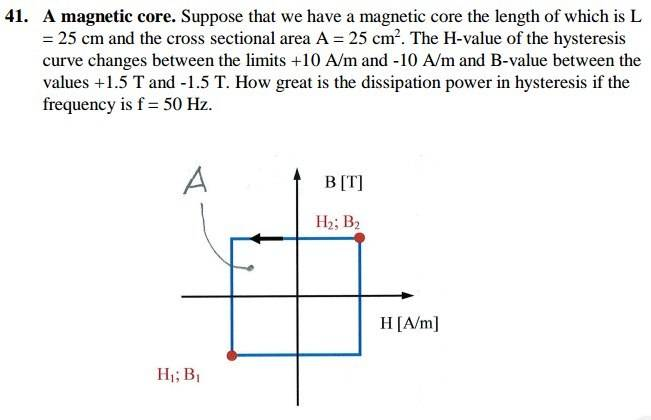# Is my solution correct? (Electromagnetism problem)

• loki4000

## Homework StatementH1=10
H2=-10
B1=1.5
B2=-1.5

## Homework Equations

(change)Uloop = (change)H2*B2 + (change)H1*B1

## The Attempt at a Solution

=(H1-H2)(B2-B1)
=(10-(-10))(-1.5-1.5)
=-60 j/m^3

You're on the right track but you have computed the energy dissipated in 1 cycle (but with the wrong units). Now convert the energy per cycle to the time rate of energy dissipation.

You're on the right track but you have computed the energy dissipated in 1 cycle (but with the wrong units). Now convert the energy per cycle to the time rate of energy dissipation.

So, it sould be something like ((H1-H2)(B2-B1))*f ?

Where did I go wrong with units?
Is it j/m^2 due to area (not volume)?

You're on the right track but you have computed the energy dissipated in 1 cycle (but with the wrong units). Now convert the energy per cycle to the time rate of energy dissipation.

So, it should be something like ((H1-H2)(B2-B1))*f ?
Yes. A lot like! :-)
Where did I go wrong with units?
Is it j/m^2 due to area (not volume)?
You didn't go wrong. I did. The per-cycle energy dissipation units are J m-3 just as you said. Except the final answer is of course in J sec-1m-3.

Yes. A lot like! :-)

You didn't go wrong. I did. The per-cycle energy dissipation units are J m-3 just as you said. Except the final answer is of course in J sec-1m-3.